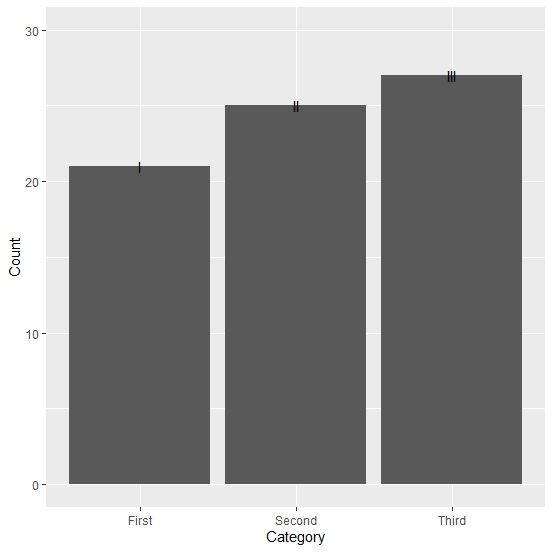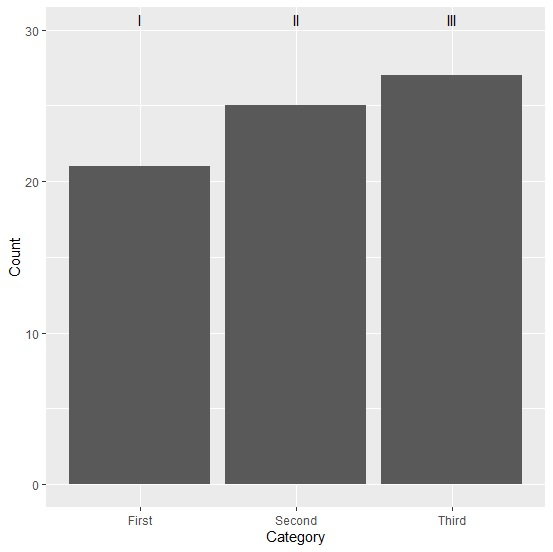# How to align the text horizontally in a bar plot created by using ggplot2 in R?

To align the text horizontally in a bar plot created by using ggplot2 in R, we can follow the below steps −

• First of all, create a data frame.

• Then, create the bar plot using ggplot2 with text displayed on each bar.

• After that, create the same bar plot with text aligned horizontally.

## Create the data frame

Let’s create a data frame as shown below −

Live Demo

Category<-c("First","Second","Third")
Count<-c(21,25,27)
df<-data.frame(Category,Count)
df

On executing, the above script generates the below output(this output will vary on your system due to randomization) −

## Output

 Category Count
1 First    21
2 Second   25
3 Third    27

## Create the bar plot with text displayed on each bar

Using annotate function to create the bar plot with text displayed on each bar −

Category<-c("First","Second","Third")
Count<-c(21,25,27)
df<-data.frame(Category,Count)
library(ggplot2)
ggplot(df,aes(Category,Count))+geom_bar(stat="identity")+scale_y_continuous(limits=c
(0,30))+annotate("text",x=1:3,y=c(21,25,27),label=c("I","II","III"))

## Output## Create the bar plot with text displayed on each bar horizontally

Using annotate function to create the bar plot with text displayed on each bar horizontally by setting y values to Inf −

Category<-c("First","Second","Third")
Count<-c(21,25,27)
df<-data.frame(Category,Count)
library(ggplot2)
ggplot(df,aes(Category,Count))+geom_bar(stat="identity")+scale_y_continuous(limits=c
(0,30))+annotate("text",x=1:3,y=Inf,vjust=1.5,label=c("I","II","III"))

## Output